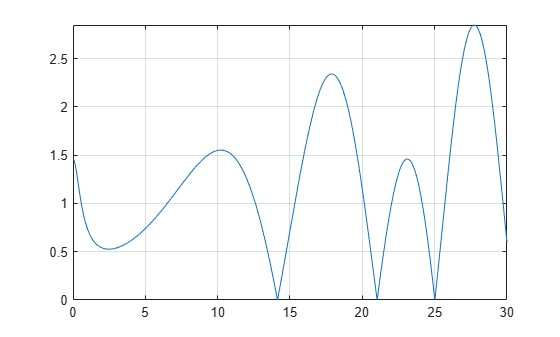# zeta

Riemann zeta function

## Syntax

``zeta(z)``
``zeta(n,z)``

## Description

example

````zeta(z)` evaluates the Riemann zeta function at the elements of `z`, where `z` is a numeric or symbolic input.```

example

````zeta(n,z)` returns the `n`th derivative of `zeta(z)`.```

## Examples

### Find Riemann Zeta Function for Numeric and Symbolic Inputs

Find the Riemann zeta function for numeric inputs.

`zeta([0.7 i 4 11/3])`
```ans = -2.7784 + 0.0000i 0.0033 - 0.4182i 1.0823 + 0.0000i 1.1094 + 0.0000i```

Find the Riemann zeta function symbolically by converting the inputs to symbolic objects using `sym`. The `zeta` function returns exact results.

`zeta(sym([0.7 i 4 11/3]))`
```ans = [ zeta(7/10), zeta(1i), pi^4/90, zeta(11/3)]```

`zeta` returns unevaluated function calls for symbolic inputs that do not have results implemented. The implemented results are listed in Algorithms.

Find the Riemann zeta function for a matrix of symbolic expressions.

```syms x y Z = zeta([x sin(x); 8*x/11 x + y])```
```Z = [ zeta(x), zeta(sin(x))] [ zeta((8*x)/11), zeta(x + y)]```

### Find Riemann Zeta Function for Large Inputs

For values of `|z|>1000`, `zeta(z)` might return an unevaluated function call. Use `expand` to force `zeta` to evaluate the function call.

```zeta(sym(1002)) expand(zeta(sym(1002)))```
```ans = zeta(1002) ans = (1087503...312*pi^1002)/15156647...375 ```

### Differentiate Riemann Zeta Function

Find the third derivative of the Riemann zeta function at point `x`.

```syms x expr = zeta(3,x)```
```expr = zeta(3, x)```

Find the third derivative at `x = 4` by substituting `4` for `x` using `subs`.

`expr = subs(expr,x,4)`
```expr = zeta(3, 4)```

Evaluate `expr` using `vpa`.

`expr = vpa(expr)`
```expr = -0.07264084989132137196244616781177 ```

### Plot Zeros of Riemann Zeta Function

Zeros of the Riemann Zeta function `zeta(x+i*y)` are found along the line `x = 1/2`. Plot the absolute value of the function along this line for `0<y<30` to view the first three zeros.

```syms y fplot(abs(zeta(1/2+1i*y)),[0 30]) grid on```## Input Arguments

collapse all

Input, specified as a number, vector, matrix or multidimensional array, or a symbolic number, variable, vector, matrix, multidimensional array, function or expression.

Order of derivative, specified as a nonnegative integer.

collapse all

### Riemann Zeta Function

The Riemann zeta function is defined by

`$\zeta \left(z\right)=\sum _{k=1}^{\infty }\frac{1}{{k}^{z}}$`

The series converges only if the real part of `z` is greater than 1. The definition of the function is extended to the entire complex plane, except for a simple pole `z = 1`, by analytic continuation.

## Algorithms

The following exact values are implemented.

• `$\zeta \left(0\right)=-\frac{1}{2}$`

• `$\zeta \left(1,0\right)=-\frac{\mathrm{log}\left(\pi \right)}{2}-\frac{\mathrm{log}\left(2\right)}{2}$`

• $\zeta \left(\infty \right)=1$

• If $z<0$ and `z` is an even integer, $\zeta \left(z\right)=0.$

• If $z<0$ and `z` is an odd integer

`$\zeta \left(z\right)=-\frac{\mathrm{bernoulli}\left(1-z\right)}{1-z}$`

For $z<-1000$, `zeta(z)` returns an unevaluated function call. To force evaluation, use `expand(zeta(z))`.

• If $z>0$ and `z` is an even integer

`$\zeta \left(z\right)=\frac{{\left(2\pi \right)}^{z}|\mathrm{bernoulli}\left(z\right)|}{2z!}$`

For $z>1000$, `zeta(z)` returns an unevaluated function call. To force evaluation, use `expand(zeta(z))`.

• If $n>0$, $\zeta \left(n,\infty \right)=0.$

• If the argument does not evaluate to a listed special value, `zeta` returns the symbolic function call.

## Version History

Introduced before R2006a